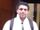## Join datasets by group in sql

How to implement the following in proc sql?

``````proc sort data = set1; by x, y; run;
proc sort data = set2; by x, y; run;

data set3;
merge set1 (in = a) set2 (in = b);
by x y;
if a and not b;
run;``````

I am trying to do this by group, and the group identifier is commonly available in set1 and set2.

9 REPLIES 9

## Re: Join datasets by group in sql

Are there variables in common between set1 and set2 other than X and Y?

Data step merge will "update" common variable other than the by variables and if you need/want that behavior we need to know.

BTW your sort syntax is incorrect. No commas in a BY statement

## Re: Join datasets by group in sql

Yes, there are common variables between the two sets. Let's call them variable z. In the sql, set2 is only used to kick out observations in set1.
You are right that my proc sort should not include commas in the by statement.

## Re: Join datasets by group in sql

Left or Right join.

See this previous questions for a good diagram (bottom) and reference to a paper that has more details.
https://communities.sas.com/t5/SAS-Procedures/Proc-SQL-equiv-of-Merge-If-A-or-B/td-p/255720

@xyxu wrote:

How to implement the following in proc sql?

``````proc sort data = set1; by x, y; run;
proc sort data = set2; by x, y; run;

data set3;
merge set1 (in = a) set2 (in = b);
by x y;
if a and not b;
run;``````

I am trying to do this by group, and the group identifier is commonly available in set1 and set2.novinosrin
Tourmaline | Level 20

## Re: Join datasets by group in sql

``````proc sql;
create table set3 as
select *
from
set1 a left join set2 b
on a.x=b.x and a.y=b.y
where a.x ne b.x and a.y ne b.y;
quit;``````

Note: I used * in select clause coz I am lazy to type and that will throw a warning because of ambiguity. However, you could type the list of variables that you need appropriately.

## Re: Join datasets by group in sql

Why should we use "and" in the where clause, instead of "or" ? My goal is to find all obs in set1 that does not have any match in set2 in terms of a pair of x and y.novinosrin
Tourmaline | Level 20

## Re: Join datasets by group in sql

"My goal is to find all obs in set1 that does not have any match in set2 in terms of a pair of x and y."--

@xyxu  Yeah OR is just fine too

on a.x=b.x and a.y=b.y
where a.x ne b.x or a.y ne b.y;

## Re: Join datasets by group in sql

``````proc sql;
create table set3 as
select t1.*
from set1 t1 left join set2 t2
on t1.x = t2.x and t1.y = t2.y
where missing(t2.x) or missing(t2.y)
;
quit;``````

## Re: Join datasets by group in sql

I  suggest you stick with data step merge (in comparison to proc SQL).

The already have a  code that  needs some modifications as follows and you will be nearing your goal.
As suggested by @ballardw  make corrections to the sort syntax.

In addition rename the common variables (other than by variables) in one of the datasets.

You may have to do home post processing to the merged dataset to get exactly what you want,

but you can easily achieve your objective of purging the rows of dataset2 from dataset1.

Discussion stats
• 9 replies
• 428 views
• 4 likes
• 7 in conversation